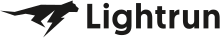• Dev Observability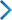• Product• Pricing
• Docs
• Resources• Blog
• Company• Debug Wordle# Stuck on an issue?

Lightrun Answers was designed to reduce the constant googling that comes with debugging 3rd party libraries. It collects links to all the places you might be looking at while hunting down a tough bug.

And, if you’re still stuck at the end, we’re happy to hop on a call to see how we can help out.

## foreach_i

See original GitHub issue

### Issue Description

I’d like to propose a new util: `foreach_i`.

## Motivation

You know how JS’s `Array.map` also passes the element index as a 2nd parameter to the function?

``````> ['a', 'b', 'c'].map((x, i) => `Element \${i} is \${x}`)
[ 'Element 0 is a', 'Element 1 is b', 'Element 2 is c' ]
``````

That’s exactly what I’m trying to do here. The only difference is that the index would be passed as the 1st param:

``````def test_foreach_i():

r = ['a', 'b', 'c'] > (pipe
| foreach_i(lambda i, x: f'Element {i} is {x}')
| list
)

assert r == [ 'Element 0 is a'
, 'Element 1 is b'
, 'Element 2 is c'
]
``````

## (Naïve) Implementation

``````from pipetools.utils import foreach, as_args
from typing import Callable, TypeVar

A = TypeVar('A')
B = TypeVar('B')

def foreach_i(f: Callable[[int, A], B]):

return enumerate | foreach(as_args(f))
``````

The same could be done for `foreach_do`.

### Issue Analytics

• State: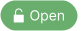• Created 7 months ago

####Top GitHub Comments

1reaction
0101commented, Jul 1, 2022

I see. Technically, you can instead do:

``````tds = tr > enumerate | foreach((X, X | colWidth.__getitem__))
``````

I think what we could do is detect if a two-parameter function is being passed in and then pass two separate parameters - and otherwise pass in the tuple so things like `foreach_i("Element {0} is {1}")` still work as expected.

0reactions
tfgacommented, Jun 29, 2022

Not really.

Every once in a while I find myself in a situation where I want to do a “foreach with index”. The particular piece of code that prompted me to write this issue was:

``````# (i, td) => (td, colWidth[i])
def pairWithWidth(i, td): return td, colWidth[i]

tds = tr >  (pipe
| enumerate
| foreach(as_args(pairWithWidth))
| ' '.join
)
``````

With `foreach_i`, it would become:

``````# (i, td) => (td, colWidth[i])
def pairWithWidth(i, td): return td, colWidth[i]

tds = tr >  (pipe
| foreach_i(pairWithWidth)
| ' '.join
)
``````

####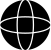Top Results From Across the Web

How do I use table.foreach() and table.foreachi()?
You pass an array and a callback function that gets called each iteration. table.foreach(tbl, function(val) ... end) table.foreachi(tbl, ...
API foreachi | WoWWiki - Fandom
Apply the function f to the elements of the table passed. On each iteration the function f is passed the index-value pair of...
Array.prototype.forEach() - JavaScript - MDN Web Docs
The forEach() method executes a provided function once for each array element.
Table Library Tutorial - lua-users wiki
foreachi () method is guaranteed to return indexed keys in order, and to skip non-index keys.) Apply the function f to the elements...
table.foreachi - Premake
table.foreachi ; Parameters​. arr is an table containing indexed values. fn is the function to call for each non-nil element. The value (not...

####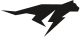Troubleshoot Live Code

Lightrun enables developers to add logs, metrics and snapshots to live code - no restarts or redeploys required.
Start Free

####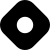Top Related Hashnode Post